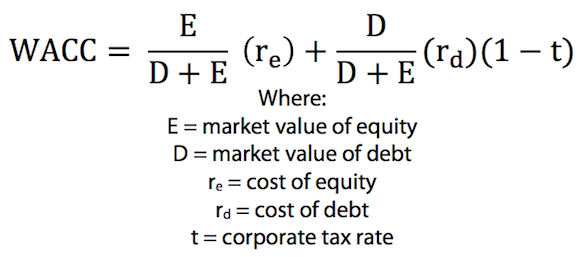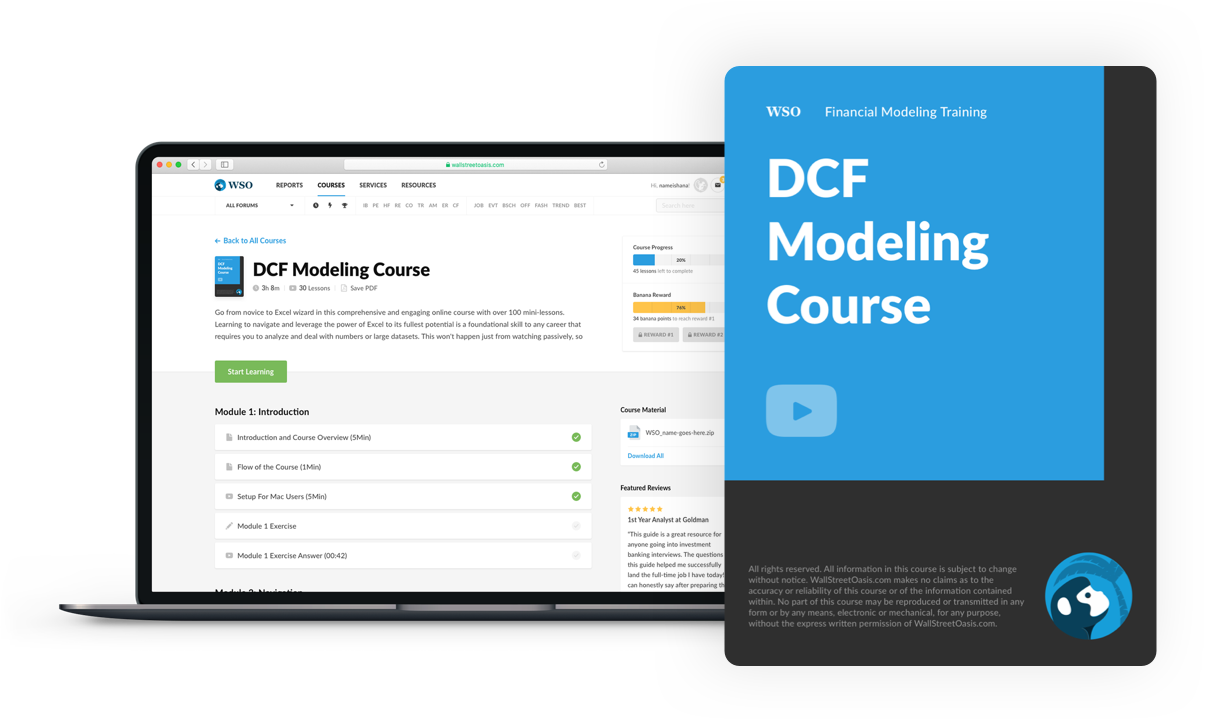Weighted Average Cost of Capital (WACC) DefinitionReviewed by

Patrick Curtis WSO Editorial Board

Expertise: Investment Banking | Private Equity

WACC, or Weighted Average Cost of Capital, is a financial metric used to measure the cost of capital to a firm. It is most usually used to provide a discount rate for a financed project, because the cost of financing the capital is a fairly logical price tag to put on the investment. WACC is used to determine the discount rate used in a DCF valuation model.

The two main sources a company has to raise money are equity and debt. WACC is the average of the costs of these two sources of finance, and gives each one the appropriate weighting.

Using a weighted average cost of capital allows the firm to calculate the exact cost of financing any project.

WACC FormulaThe formula for how to calculate WACC may seem complicated but in reality is fairly simple:

• (Percentage of finance that is equity x Cost of Equity) + (Percentage of finance that is debt x Cost of Debt) x (1 – Tax Rate)Module 1: Introduction

Module 2: Valuation: The Big Picture

Module 3: Enterprise Value & Equity Value Practice

Module 5: Trading Comps: The Setup

Module 10: Trading Comps: Benchmarking and Outputs

Module 11: Precedent Transactions: Introduction

Module 12: Precedents: The Setup

Module 13: Spreading Tiffany & LVMH

Module 16: Spreading Jimmy Choo & Michael Kors

Module 17: Spreading Dickies & VF

Module 18: Valuation Wrap-Up

Module 19: Bonus: Non-GAAP Practice

Related Terms